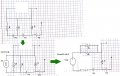# Finding current using Thevenin

#### gbox

Joined Dec 29, 2015
42So I tried to find R thevenin:But what should I do with the short circuit? are those 2 resistors connected in parallel or series?

How do I find V thevenin?

#### MrAl

Joined Jun 17, 2014
10,889
Hi,

Last time i checked, a short in theory is zero ohms (0 ohms) and 0 ohms in parallel with any other resistance is still zero ohms.

[See attachment where the first main step has been illustrated]

To find Vth, you could start at the left side and work your way across, after removing that top resistor which is shorted out. Enumerate the voltage sources from left to right as V1, V2, and V3. The resistances appear to be all of the same value so we dont have to enumerate them, but if they were different values or could be different then we would have to enumerate them also. The voltage sources have no label so they might be all the same voltage too, but we'll enumerate them anyway for clarity in the procedure that follows.

Starting with the first resistor and voltage source V1 on the left, convert that into a current source:
I1=V1/R
We then have a current source I1 in parallel with two resistors R and R, so we have R/2 in parallel with I1.
We can therefore convert this back into a voltage source in series with a resistor where:
V1x=I1*R/2
and the series resistor is R/2.
Now we have two voltage sources in series with a resistor R/2. The total voltages is:
V2x=V1x+V2
and the series resistance is R/2. Thus the circuit is already simplified into just two sources and two resistors.

Next, we do the same thing as before. Convert the new source V2x into a current source:
I2=V2x/(R/2)=V2x*2/R
with parallel resistance R/2.
This gives us two resistors in parallel, one is R/2 and the other is R, so the combined resistance is R/3.
Converting back to a voltage source, we have current I2 and resistance R/2 so we have voltage:
V3x=I2*R/3
and series resistance:
R/3.

This again gives us two voltage sources in series with one resistor where the voltage is:
Vth=V3x+V3
and the series resistance is:
Rth=R/3.

If you trace the calculations for Vth back, you'll fiind you can easily eliminate all the current sources by representing them as a voltage divided by a resistance. I'll leave that to you. You can also carry all the calculations over in each step so you dont have to back track later, which means the current sources will carry as some V over some R for each step.
Try that and see if you can get the result now.

Note again that we just used the two rules:
1. A voltage source in series with a resistance is equivalent to a current source in parallel with a resistance.
2. A current source in parallel with a resistance is equivalent to a voltage source in series with a resistance.

By converting back and forth we can simplify circuits, usually following the circuit from left to right.Last edited:

#### RBR1317

Joined Nov 13, 2010
712
A simpler method to find the Thevenin voltage is to write the node equation for the Thevenin circuit, realizing there is a single supernode where all the voltages are constant offsets from the Thevenin voltage. The only unknown is Vth so only one equation is necessary. Note the supernode equation was solved using Maple.

Mods Edit: